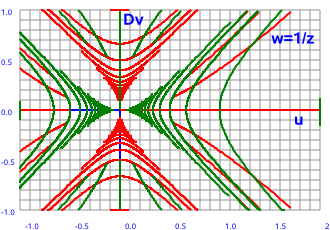# Maths - Inverse Of Double Numbers

To calculate the inverse value (1/z) we multiply the top and bottom by the conjugate which makes the denominator a real number.

z plane   w plane-->

w=1/zhow this plot was produced.

Let the components of the input and output planes be:

z = x + D y and w = u + D v

In this case w = 1/z

so:

w = 1/(x + D y)

As usual, we evaluate the inverse by multiplying top and bottom by the conjugate:

w = (x - D y)/(x + D y)(x - D y)

w = (x - D y)/(x² - y²)

so the u and v components are:

u = x /(x²-y²)
v = -y /(x²-y²)